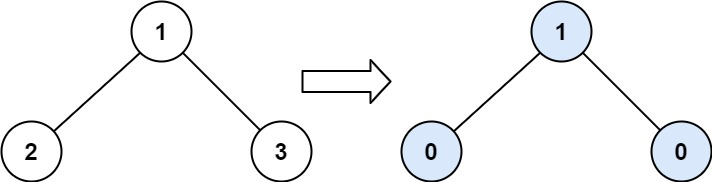# Leetcode 563 - Binary tree tilt

Note:

• DFS all the way! I hate iterative traverses.
• Base case: we are looking for num, so apparently when node is null, we should return 0.
• Return |leftSum - rightSum|.

Question:

Given the root of a binary tree, return the sum of every tree node’s tilt.

The tilt of a tree node is the absolute difference between the sum of all left subtree node values and all right subtree node values. If a node does not have a left child, then the sum of the left subtree node values is treated as 0. The rule is similar if there the node does not have a right child.

Example:Code: